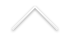Data: 29/08/2018 - Quarta-feira
Horário: 11:00
Local: IM-UFRJ, CT, sala C119

Palestrante: Dominik Kwietniak (Jagiellonian University in Krakow)

Resumo: We study the Borel complexity of sets of normal numbers in several numeration systems. Taking a dynamical point of view, we offer a unified treatment for continued fraction expansions and base b-expansions, and their various generalisations: generalised Luroth series expansions and beta-expansions. In fact, we consider subshifts over a countable alphabet generated by all possible expansions of numbers in [0,1). Then normal numbers correspond to generic points of shift-invariant measures. It turns out that for these subshifts the set of generic points for a shift-invariant probability measure is precisely at the third level of the Borel hierarchy (it is a so called pi^0_3-complete set, meaning that it is a countable intersection of F_sigma-sets, but it is not possible to write it as a countable union of G_delta-sets). We also solve Sharkovsky--Sivak problem on Borel complexity of the basin of statistical attraction. The crucial dynamical feature we need is a feeble form of specification. All expansions named above generate subshifts with this property. Hence sets of normal numbers under consideration are pi^0_3-complete. The talk is based on a joint work with: Dylan Airey, Steve Jackson, and Bill Mance.

Data: 24/05/2018
Hora: 13:15
Local: Sala B106-b

Palestrante: Charles López Vereau

Título:Método de Moser

Resumo: Nesta palestra apresentaremos a importˆancia do m´etodo de Moser na geometria, como ele atua nas demonstra¸c˜oes dos Teoremas de Formas Normais, que tamb´em s˜ao chamados Teoremas de Rigidez Local. Nos centraremos em trˆes destes Teoremas, os quais s˜ao o Teorema de Darboux para Formas Simpl´eticas, o Teorema de Darboux para Formas de Contato e o Teorema da Vizinhan¸ca Lagrangiana.

Data: 03/05/2018
Hora: 13:00
Local: Sala C119
Palestrante: Marcos Cossarini (IMPA)

Título: Discrete geometry of surfaces towards the filling area conjecture

Resumo: In 1983, Gromov conjectured that the hemisphere has smallest area among Riemannian surfaces that fill isometrically a circle of given length. We discuss the history of the problem and present a discrete version, where a cycle graph of length 2n is filled isometrically by a combinatorial square-celled surface with the least possible number of cells, conjectured to be n(n-1)/2. The discrete problem is equivalent to Gromov's filling area conjecture, as extended by Ivanov to admit filling surfaces with self-reverse Finsler metrics, because every such continuous metric can be replaced by a square-celling whose lengths and areas approximate the original values as precisely as desired (quasi-isometrically with arbitrarily good additive and multiplicative constants).